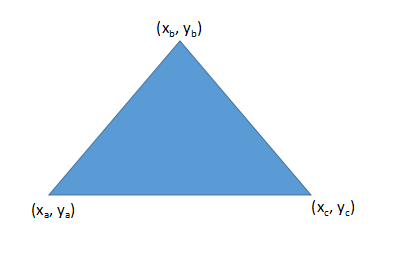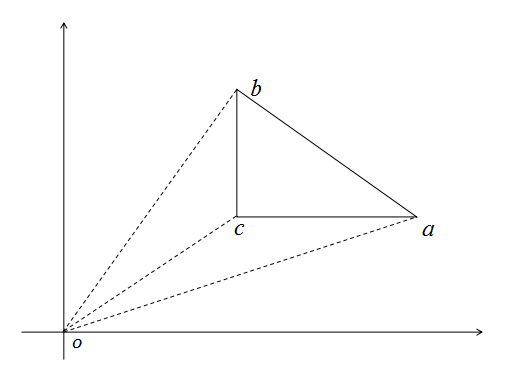• 不规则多边形区域的面积计算算法最近在写一个显微图像分析处理方面的程序，里面有一个功能是计算一个不规则的多边形区域的面积。因此花了点时间研究这个算法该如何写。研究了一番之后，算是找到了个比较靠谱的算法。...
不规则多边形区域的面积计算算法

最近在写一个显微图像分析处理方面的程序，里面有一个功能是计算一个不规则的多边形区域的面积。因此花了点时间研究这个算法该如何写。研究了一番之后，算是找到了个比较靠谱的算法。这里就简单的写写自己的研究成果。

解决这个问题，需要点线性代数和矢量运算方面的知识。以下图为例，设三角形的三个顶点为 a,b,c$a, b, c$，坐标分别为 (xa,ya),(xb,yb),(xc,yc)$(x_a, y_a), (x_b,y_b),(x_c, y_c)$。那么三角形的面积可以用行列式来表示：

S=12∣∣∣∣∣xaxbxcyaybyc111∣∣∣∣∣
S = \frac{1}{2}
\begin{vmatrix}
x_a &  y_a & 1 \\
x_b & y_b & 1 \\
x_c & y_c & 1
\end{vmatrix}

需要特别注意的是这里 a,b,c$a, b, c$ 的排列，像图中这样 a,b,c$a, b, c$ 顺时针排列的，计算出的 S$S$ 是负数，需要再取个绝对值。如果 a,b,c$a, b, c$ 是逆时针排列的，计算出的结果直接就是正的。

面积也可以用矢量的叉积来表示：
S=12∥∥∥ab→×ac→∥∥∥
S =\frac{1}{2} \left\| \vec{ab} \times \vec{ac} \right\|

这里的 ∥⋅∥$\| \cdot \|$ 表示求矢量的模。当然也可以不求矢量的模，我们知道矢量叉积是另一个矢量，方向满足右手定则。因此 a,b,c$a, b, c$ 顺时针排列的，计算出的 S$S$ 是负方向的，a,b,c$a, b, c$ 是逆时针排列的，计算出的结果直接就是正的。

当 a$a$ 点的坐标为 (0,0)$(0, 0)$ 时，上面的两个式子都可以简化为:
S=12∣∣∣xbxcybyc∣∣∣=12∥xbyc−xcyb∥S =\frac{1}{2} \begin{vmatrix}  x_b & y_b\\ x_c & y_c \end{vmatrix}=\frac{1}{2} \|x_b y_c - x_c y_b\|

当三角形的三个顶点都不在原点时，我们也可以借助原点来计算。从上图中我们可以看出。
Sabc=Soab−Socb−Soac
S_{abc} = S_{oab} - S_{ocb} - S_{oac}

用行列式表示的话可以写为：
Sabc=12∣∣∣xaxbyayb∣∣∣−12∣∣∣xcxbycyb∣∣∣−12∣∣∣xaxcyayc∣∣∣=12∣∣∣xaxbyayb∣∣∣+12∣∣∣xbxcybyc∣∣∣+12∣∣∣xcxaycya∣∣∣
\begin{eqnarray}
S_{abc} =  \frac{1}{2}
\begin{vmatrix}  x_a & y_a\\
x_b & y_b \end{vmatrix} -
\frac{1}{2} \begin{vmatrix}  x_c & y_c\\
x_b & y_b \end{vmatrix} -
\frac{1}{2} \begin{vmatrix}  x_a & y_a\\
x_c & y_c \end{vmatrix} \\
=\frac{1}{2}
\begin{vmatrix}  x_a & y_a\\
x_b & y_b \end{vmatrix} +
\frac{1}{2} \begin{vmatrix}  x_b & y_b\\
x_c & y_c \end{vmatrix} +
\frac{1}{2} \begin{vmatrix}  x_c & y_c\\
x_a & y_a \end{vmatrix}
\end{eqnarray}

或者用矢量的叉积来写：

Sabc=12∥∥∥oa→×ob→∥∥∥−12∥∥∥oc→×ob→∥∥∥−12∥∥oa→×oc→∥∥=12∥∥∥oa→×ob→+ob→×oc→+oc→×oa→∥∥∥
\begin{eqnarray}
S_{abc} = \frac{1}{2} \left\| \vec{oa} \times \vec{ob} \right\| -
\frac{1}{2} \left\| \vec{oc} \times \vec{ob} \right\|  -
\frac{1}{2} \left\| \vec{oa} \times \vec{oc} \right\| \\
= \frac{1}{2} \left\| \vec{oa} \times \vec{ob} +  \vec{ob} \times \vec{oc} + \vec{oc} \times \vec{oa} \right\|
\end{eqnarray}

无论是用行列式来表示还是矢量叉积表示，式子都变得非常规矩，很容易用程序代码实现。

多边形区域的面积计算和这个计算的思想是一样的。每个线段与坐标原点都能围成一个三角形，这些三角形的“面积”之和就是多边形区域的面积。这里的面积打了引号，因为这些三角形的顶点排列顺序不同时，算出的面积可以是负值。

设多边形的各个顶点按逆时针排列分别是 v1,v2,⋯vn$v_1, v_2, \cdots v_n$。顶点坐标依次是 (x1,y1),(x2,y2),⋯(xn,yn)$(x_1, y_1), (x_2, y_2) ,\cdots (x_n, y_n)$，那么面积可以写为：

S=12(∣∣∣x1x2y1y2∣∣∣+∣∣∣x2x3y2y3∣∣∣+⋯+∣∣∣xn−1xnyn−1yn∣∣∣+∣∣∣xnx1yny1∣∣∣)
S = \frac{1}{2} \left(
\begin{vmatrix}  x_1 & y_1\\
x_2 & y_2 \end{vmatrix} +
\begin{vmatrix}  x_2 & y_2\\
x_3 & y_3 \end{vmatrix} + \cdots +
\begin{vmatrix}  x_{n-1} & y_{n-1}\\
x_n & y_n \end{vmatrix} +
\begin{vmatrix}  x_n & y_n\\
x_1 & y_1 \end{vmatrix}
\right)

如果顶点的顺序是顺时针的，那么这么求出的面积就是负的，还要再取个负号。

至此，多边形面积计算问题就算解决了。
展开全文• 最近开发过程中遇到了这个问题，费了好大劲才搞定，贴出来跟大家分享 假设lpVertex1[]里存放着多边形1顶点，nVertexCount1为多边形1顶点数 lpVertex2[]里存放着多边形2...//创建多边形区域1 CRgn poly1; poly1...
最近开发过程中遇到了这个问题，费了好大的劲才搞定，贴出来跟大家分享           假设lpVertex1[]里存放着多边形1的顶点，nVertexCount1为多边形1的顶点数      lpVertex2[]里存放着多边形2的顶点，nVertexCount2为多边形2的顶点数          //创建多边形区域1          CRgn   poly1;          poly1.CreatePolygonRgn(lpVertex1,nVertexCount1,ALTERNATE);          delete[]   lpVertex1;          //创建多边形区域2          CRgn   poly2;          poly2.CreatePolygonRgn(lpVertex2,nVertexCount2,ALTERNATE);          delete[]   lpVertex2;               //获取相交的多边形区域          CRgn   IntersectRgn;          IntersectRgn.CreateRectRgn(0,0,0,0);          int   nIntersectResult   =   IntersectRgn.CombineRgn(   &poly1,&poly2,RGN_AND);          if(nIntersectResult==ERROR)                {      AfxMessageBox("多边形求交出错");      return   0;                }            //没有相交            if(nIntersectResult==NULLREGION)                    return   0;            /***********精华部分到了************/              //计算相交部分的面积              Region   intersectRegion((HRGN)IntersectRgn);              Matrix   matrix;              Rect*   rects   =   NULL;              int   count   =   0;                     CClientDC   dc(this);              Graphics   graphics(   dc.GetSafeHdc()   );              graphics.GetTransform(&matrix);              count   =   intersectRegion.GetRegionScansCount(&matrix);              rects   =   (Rect*)malloc(count*sizeof(Rect));              intersectRegion.GetRegionScans(&matrix,   rects,   &count);                           double   dbTotalArea=0;              for(int   j   =   0;   j   <   count;   ++j)                  dbTotalArea +=(rects[j].GetBottom()-rects[j].GetTop())*   (rects[j].GetRight()-rects[j].GetLeft());                   free(rects);              graphics.ReleaseHDC(dc.GetSafeHdc());              //返回面积值              return   dbTotalArea;           该方法不仅可以计算多边形之间相交部分的面积，而且可以算圆和多边形的相交面积，甚至是任意图形相交部分的面积。      在使用该方法的时候，用到了GDI+的相关类Region,相关资料请查看MSDN。      顺便说一句：之前在网上找相关算法，找了大半天才找到一个像样的，编好程序以后      发现该算法有漏洞，白编了，呵呵，组长叫我好好看看GDI的Region类，结果给我找到了这个方法，所得：有些东西MFC里都有现成的(特别是图形相关)，用起来很方便。找算法往往又费劲，又得担心算法的正确性，不怎么好。
展开全文• 在AngusClipper与其他库基准测试中，他有一个多边形区域计算方法 . 我用它并修改了他椭圆方法 . 结果如下：void Ellipse2Poly(double theta, double A1, double B1, double H1, double K1, Poly& p){const...
在Angus的Clipper与其他库的基准测试中，他有一个多边形区域计算方法 . 我用它并修改了他的椭圆方法 . 结果如下：void Ellipse2Poly(double theta, double A1, double B1, double H1, double K1, Poly& p){const double pi = 3.1415926535898, tolerance = 0.125;const int n = 30;double step = pi/(double)n;double a = A1; // Long semi-axis lengthdouble b = B1; // short semi-axis lengthdouble xc = H1; // current X positiondouble yc = K1; // current Y positiondouble sintheta = sin(theta);double costheta = cos(theta);double t = 0;p.resize(2*n+1);for (int i = 0; i < 2*n+1; i++){p[i].x = xc + a*cos(t)*costheta - b*sin(t)*sintheta;p[i].y = yc + a*cos(t)*sintheta + b*sin(t)*costheta;t += step;}}面积计算是：double OverlapArea(Poly poly1, Poly poly2){Poly clip;Polys polys_poly1, polys_poly2, polys_clip;polys_poly1.resize(1);polys_poly2.resize(1);polys_clip.resize(1);polys_poly1 = poly1;polys_poly2 = poly2;polys_clip = clip;Polygons clipper_polys_poly1, clipper_polys_poly2, clipper_polys_clip;LoadClipper(clipper_polys_poly1,polys_poly1);LoadClipper(clipper_polys_poly2,polys_poly2);ClipType op = ctIntersection;Clipper cp;cp.AddPolygons(clipper_polys_poly1,ptSubject);cp.AddPolygons(clipper_polys_poly2,ptClip);cp.Execute(op,clipper_polys_clip,pftEvenOdd,pftEvenOdd);if(clipper_polys_clip.size() != 0){UnloadClipper(polys_clip,clipper_polys_clip);return Area(polys_clip);}elsereturn 0.0;}它比_1384765慢，但非常稳定 . 我一夜之间运行它2 * 10 ^ 5步，它没有崩溃 . 花了6小时12分钟，而Boost需要大约4小时，但我很高兴 .EDIT:我重写了其他一些功能，所以比较对Clipper不公平！对于相同的配置和100K时间步长，Clipper在2小时36分钟内完成了操作，我觉得非常好！ Boost将在大约2小时15分钟内完成相同的操作，所以我发现它们在这些长跑中非常可比！
展开全文• Does anyone know how to calculate the area in common between 2 or more polygons in R? I would like to have the output of such a calculation be the coordinates of a new polygon for that area of overlap...Does anyone know how to calculate the area in common between 2 or more polygons in R? I would like to have the output of such a calculation be the coordinates of a new polygon for that area of overlap.Cheers解决方案EDIT: these days I would use the 'intersect', 'cover', 'erase', 'union' and related functions in the 'raster' package. They do the hard work to keep the top-level object and attributes.ORIG:You could use the rgeos package with its gIntersection function. Successive calls between pairs and resulting intersections will get you there. Seelibrary(rgeos)?gIntersectionYou will need to get into the structure of "SpatialPolygons" in the sp package to get the final coordinates. See the vignette("sp").
展开全文• 任意多边形面积计算根据坐标直接按公式计算。---------------------------------------------------------------这是一个问题早已经解决经典问题假定多边形ｎ个顶点坐标依次是(x1,y1),...(xn,yn)如果ｎ个顶点是逆...
• 基于GPU任意多边形相交面积计算方法高艺1,2,罗健欣1,裘杭萍1,唐斌1,吴波1【摘要】一直以来，任意多边形相交面积的高效计算都是地理信息系统中空间分析算法研究重点。文中提出了一种基于GPU栅格化多边形相交...
• 问题描述最近在做微信小程序开发，需求中要突出显示山西省地图。...map组件有一个polygons属性可以绘制一个多边形，并且可以填充颜色。2.看文档之后发现需要一个经纬度数组。这个经纬度数组还得...倒计时小程序桌面显示
• 之前尝试过很多任意多边形面积计算，都有不同局限性，直到使用了这个公式后，问题就解决了，感谢原作者分享。//堰槽坐标定义typedefstruct{doublex;doubley;}WeirCoorType;//任意多边形面积计算doubleCLASS_...
• 注意是，求出是有向面积，有可能是负数。#include #include #include #include #include #include #include #include #include #include #include #include #define CL(arr, val) memset(arr, val, sizeof(arr.....
• 题目：输入一个点列，顺次连接成一个封闭多边形，计算多边形的面积 分析：方法一，计算面积可以考虑定积分的形式，定积分有正有负，顺次求和，重复部分相互抵消，最后剩下的总面积的绝对值就是多边形的面积。 ...float struct null 算法
• AreaOnAreaOverlayer通常用于三个主要操作：(1)解构重叠多边形以产生交叉和差异，(2)比较区域重叠的多个数据集，以及(3)基于重叠面数据的面积计算。polygon-in-polygon-overlay.fmwthistoriczones....
• 钢结构油漆面积是按照钢材实际展开面积计算钢结构中油漆有按照重量刷油项目，直接套用即可钢结构工程量计算方法及规则01、金属结构工程(一)钢屋架、钢网架(1)按设计图示尺寸以钢材重量计算，不扣除孔眼、切边、...
• 任意两个凸多边形A,B相交区域面积计算，这里采用如下两步进行计算： 一、 计算相交区域对应的多边形，记为intersect 大体分为下面几个步骤： 1.记录A位于B中所有顶点 2.记录B位于A中所有顶点 3.记录A与B每一条...
• 只要是边不相交简单多边形，也就是说，不仅凸多边形，还有各种奇形怪状多边形，都可以用格林公式求出面积。格林公式：若函数P(x,y), Q(x,y)在由一条或几条光滑曲线所围成区域D上连续，且有连续一阶偏导...
• 钢结构油漆面积是按照钢材实际展开面积计算序号钢材类别截面面积计算公式代号说明1圆钢、圆盘条、钢丝F=0.785 4d2d—外径2方钢F=a2a—边宽3圆角方钢F=a2-0.858 4r2a—边宽,r—圆角半径4六角钢F=0.866a2=2.598s2a—对...
• 素数分布波动p=sin(np)，质数间距计算、大质数寻找“锐器”—P=［n/p］ - 知乎sdsxdwd：素数分布波动p=sin(np)，质数间距计算、大质数寻找“锐器”—P=［n/p］​zhuanlan.zhihu.com1.质数向量距离等于0；n元集中最多...
• I have map. On the top of map layer have a polygon A and circle B. They are intersected each others. Any algorithm can calculate the area of intersection C ?解决方案Assuming that you're willing to ...
• geojson-area, 计算geojson多边形或者多重多边形的面积 geojson区域计算任意 GeoJSON 几何图形内的区域。用法npm install @mapbox/geojson-area示例var geojsonArea = require('@mapb
• （e.g: xx地区发生暴雪，暴雪区域多边形，给多边形中心加一个暴雪图标） 之前设计是，计算不规则多边形范围矩形bounds中心点。这个比较简单，对于一些圆，矩形，凸多边形都比较适合。但是遇到凹多边形就会...
• hold off [k,a] = convhull(xv,yv) dt = DelaunayTri Properties: Constraints: [] X: [9x2 double] Triangulation: [7x3 double] Methods, Superclasses k = 1 2 3 4 5 6 7 8 9 1 面积为 a = 4.0729 转载本文请...
• hold off [k,a] = convhull(xv,yv) dt = DelaunayTri Properties: Constraints: [] X: [9x2 double] Triangulation: [7x3 double] Methods, Superclasses k = 1 2 3 4 5 6 7 8 9 1 面积为 a = 4.0729 转载本文请...
• 在学习计算几何（至少是这篇文章）之前，你需要了解以下这些东西：平面直角坐标系向量及其运算三角剖分求面积平面直角坐标系平面直角坐标系就是笛卡尔坐标系，这一部分会在人教版七年级数学中系统地学习。...
• (2)不规则或多边形钢板，以其外接规则矩形面积计算。(3)钢网架应区分球形结点、钢板结点等连接形式。(4)计量单位为t。(二)钢托架，钢桁架(1)按设计图示尺寸以钢材重量计算。不扣除孔眼、切边、切肢重量，焊条、铆 ...
• (2)不规则或多边形钢板，以其外接规则矩形面积计算。(3)钢网架应区分球形结点、钢板结点等连接形式。(4)计量单位为t。(二)钢托架，钢桁架(1)按设计图示尺寸以钢材重量计算。不扣除孔眼、切边、切肢重量，焊条、铆 ...
• （e.g: xx地区发生暴雪，暴雪区域多边形，给多边形中心加一个暴雪图标） 之前设计是，计算不规则多边形范围矩形bounds中心点。这个比较简单，对于一些圆，矩形，凸多边形都比较适合。但是遇到凹多边形就会...google map map polygon center
• (2)不规则或多边形钢板，以其外接规则矩形面积计算。(3)钢网架应区分球形结点、钢板结点等连接形式。(4)计量单位为t。(二)钢托架，钢桁架(1)按设计图示尺寸以钢材重量计算。不扣除孔眼、切边、切肢重量，焊条、铆 ...
• 目标：计算红色区域的面积 实现的方法： 1、首先能够在鼠标点击事件、鼠标移动事件、和paint事件中实现多边形的绘制。 2、利用GraphicsPath记录多边形顶点坐标；System.Drawing.Region 记录多边形区域 3、 使用...
• 计算不规则多边形的面积、中心、重心 最近项目用到：在不规则多边形的中心点加一个图标。（e.g: xx地区发生暴雪，暴雪区域多边形，给多边形中心加一个暴雪的图标） 之前的设计是，计算不规则多边形范围矩形......